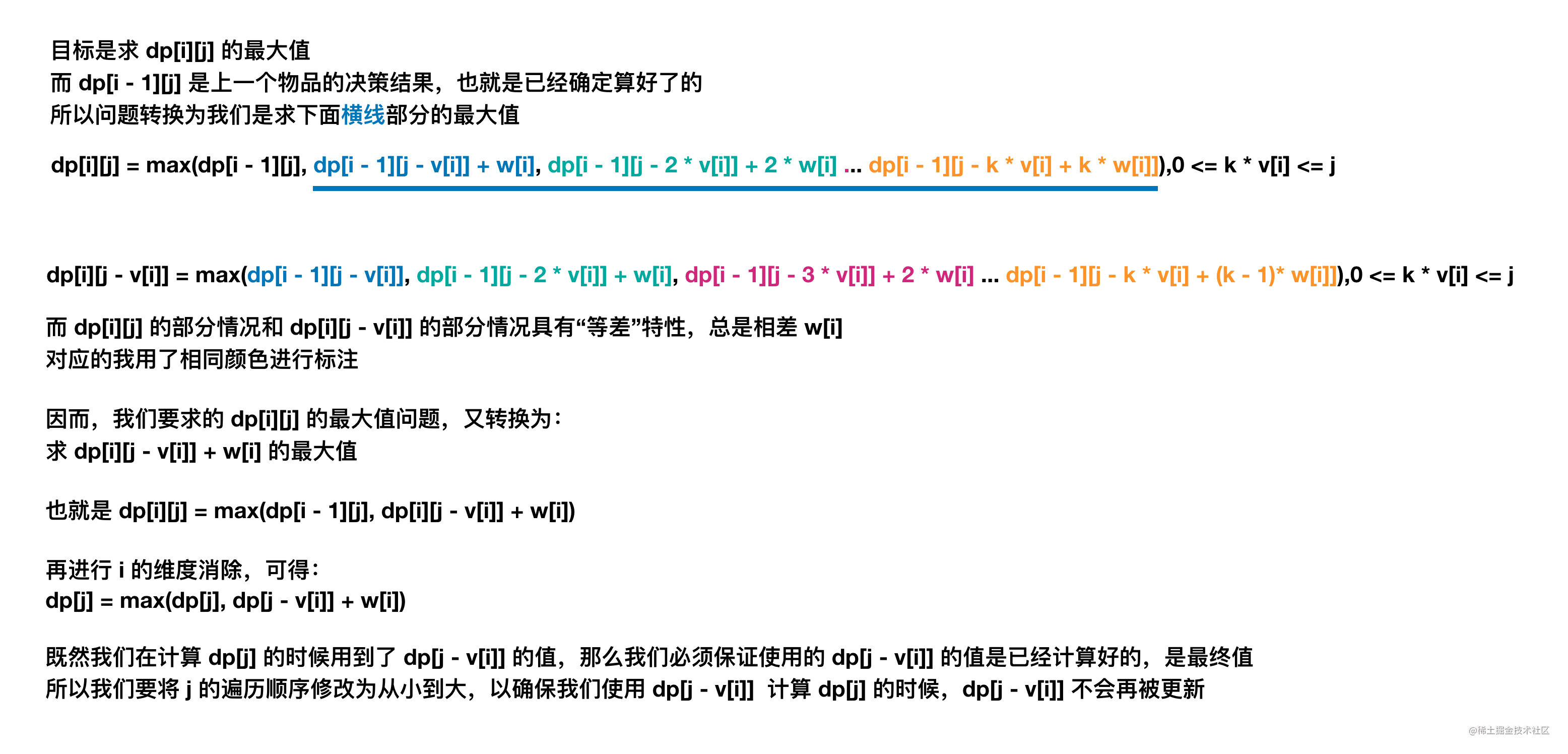# 【背包问题の第四讲】从数学角度推导「完全背包」与「01 背包」之间的遍历顺序关系

## 题目描述

$N$ 种物品和一个容量为 $C$ 的背包，每种物品都有无限件。

$i$ 件物品的体积是 $v[i]$，价值是 $w[i]$

输入: N = 2, C = 5, v = [1,2], w = [1,2]

## 常规解法

$dp[i][j]$ 代表考虑前 $i$ 件物品，放入一个容量为 $j$ 的背包可以获得的最大价值。

• 选择 0 件物品 $i$ 的最大价值，即 $dp[i-1][j]$

• 选择 1 件物品 $i$ 的最大价值，即 $dp[i-1][j-v[i]]+w[i]$

• 选择 2 件物品 $i$ 的最大价值，即 $dp[i-1][j-2*v[i]]+2*w[i]$

...

• 选择 k 件物品 $i$ 的最大价值，$dp[i-1][j-k*v[i]]+k*w[i]$

$dp[i][j] = max(dp[i - 1][j], dp[i - 1][j - k * v[i]] + k * w[i]), 0 < k*v[i] \leqslant j$

class Solution {
public int maxValue(int N, int C, int[] v, int[] w) {
int[][] dp = new int[N][C + 1];

// 先预处理第一件物品
for (int j = 0; j <= C; j++) {
// 显然当只有一件物品的时候，在容量允许的情况下，能选多少件就选多少件
int maxK = j / v;
dp[j] = maxK * w;
}

// 处理剩余物品
for (int i = 1; i < N; i++) {
for (int j = 0; j <= C; j++) {
// 不考虑第 i 件物品的情况（选择 0 件物品 i）
int n = dp[i - 1][j];
// 考虑第 i 件物品的情况
int y = 0;
for (int k = 1 ;; k++) {
if (j < v[i] * k) {
break;
}
y = Math.max(y, dp[i - 1][j - k * v[i]] + k * w[i]);
}
dp[i][j] = Math.max(n, y);
}
}
return dp[N - 1][C];
}
}

• 时间复杂度：共有 $N * C$ 个状态需要被转移，但每次转移都需要枚举当前物品的件数，最多枚举 C 次（重量为最小值 1），因此整体复杂度为 $O(N * C * C)$
• 空间复杂度：$O(N * C)$

## 滚动数组

class Solution {
public int maxValue(int N, int C, int[] v, int[] w) {
int[][] dp = new int[C + 1];

// 先预处理第一件物品
for (int j = 0; j <= C; j++) {
// 显然当我们只有一件物品的时候，在容量允许的情况下，能选多少件就选多少件
int maxK = j / v;
dp[j] = maxK * w;
}

// 处理剩余物品
for (int i = 1; i < N; i++) {
for (int j = 0; j <= C; j++) {
// 不考虑第 i 件物品的情况（选择 0 件物品 i）
int n = dp[(i - 1)&1][j];
// 考虑第 i 件物品的情况
int y = 0;
for (int k = 1 ;; k++) {
if (j < v[i] * k) {
break;
}
y = Math.max(y, dp[(i - 1)&1][j - k * v[i]] + k * w[i]);
}
dp[i&1][j] = Math.max(n, y);
}
}
return dp[(N - 1)&1][C];
}
}

• 时间复杂度：共有 $N * C$ 个状态需要被转移，但每次转移都需要枚举当前物品的件数，最多枚举 C 次（重量为最小值 1），因此整体复杂度为 $O(N * C * C)$
• 空间复杂度：$O(N * C)$

## 一维空间优化

$dp[j] = max(dp[j], dp[j - v[i]] + w[i])$

PS. 如果你不太理解上面的话，或许是因为你「还没学习」或者「有点忘记」01 背包问题，三叶强烈建议你先对 01 背包问题 进行学习/回顾。

「01 背包将容量维度「从大到小」遍历代表每件物品只能选择一件，而完全背包将容量维度「从小到大」遍历代表每件物品可以选择多次。」

$dp[i][j] = max(dp[i - 1][j], dp[i - 1][j - v[i]] + w[i], dp[i - 1][j - 2 * v[i]] + 2 * w[i],...,dp[i - 1][j - k * v[i]] + k * w[i]), 0\leqslant k*v[i] \leqslant j$

$dp[i][j]$ 所代表的含义：在容量允许的情况下，对于第 $i$ 件物品，我们可以不选，可以选 1 次，可以选 2 次，...，可以选 $k$ 次 ...

$dp[i][j - v[i]] = max(dp[i - 1][j - v[i]], dp[i - 1][j - 2 * v[i]] + w[i], dp[i - 1][j - 3 * v[i]] + 2 * w[i],...,dp[i - 1][j - k * v[i]] + (k - 1) * w[i]), 0\leqslant k*v[i] \leqslant j$• 0-1 背包问题的状态转换方程是：
$dp[i][j] = max(dp[i - 1][j], dp[i - 1][j - v[i]] + w[i])$

• 完全背包问题的状态转移方程是：
$dp[i][j] = max(dp[i - 1][j], dp[i][j - v[i]] + w[i])$

class Solution {
public int maxValue(int N, int C, int[] v, int[] w) {
int[] dp = new int[C + 1];
for (int i = 0; i < N; i++) {
for (int j = 0; j <= C; j++) {
// 不考虑第 i 件物品的情况（选择 0 件物品 i）
int n = dp[j];
// 考虑第 i 件物品的情况
int y = j - v[i] >= 0 ? dp[j - v[i]] + w[i] : 0;
dp[j] = Math.max(n, y);
}
}
return dp[C];
}
}

• 时间复杂度：共有 $N * C$ 个状态需要被转移，复杂度为 $O(N * C)$
• 空间复杂度：$O(C)$

## 总结

• 01 背包依赖的是「上一行正上方的格子」和「上一行左边的格子」。

• 完全背包依赖的是「上一行正上方的格子」和「本行左边的格子」。

## 背包问题（目录）

1. 01背包 : 背包问题 第一讲

1. 【练习】01背包 : 背包问题 第二讲

2. 【学习&练习】01背包 : 背包问题 第三讲

2. 完全背包 : 本篇

1. 【练习】完全背包 : 背包问题 第五讲

2. 【练习】完全背包 : 背包问题 第六讲

3. 【练习】完全背包 : 背包问题 第七讲

3. 多重背包 : 背包问题 第八讲

4. 多重背包（优化篇）

1. 【上】多重背包（优化篇）: 背包问题 第九讲

2. 【下】多重背包（优化篇）: 背包问题 第十讲

5. 混合背包 : 背包问题 第十一讲

6. 分组背包 : 背包问题 第十二讲

1. 【练习】分组背包 : 背包问题 第十三讲
7. 多维背包

1. 【练习】多维背包 : 背包问题 第十四讲

2. 【练习】多维背包 : 背包问题 第十五讲

8. 树形背包 : 背包问题 第十六讲

1. 【练习篇】树形背包 : 背包问题 第十七讲

2. 【练习篇】树形背包

9. 背包求方案数

1. 【练习】背包求方案数
10. 背包求具体方案

1. 【练习】背包求具体方案
11. 泛化背包

1. 【练习】泛化背包